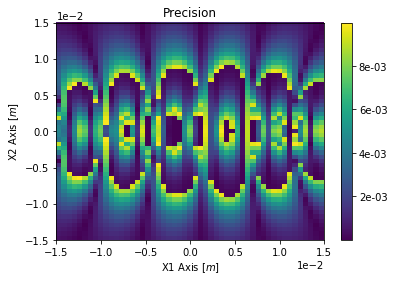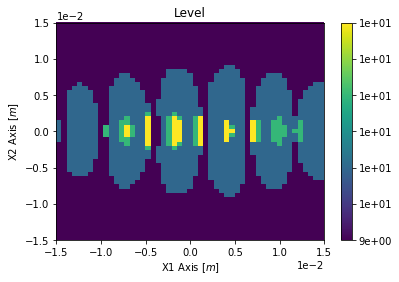# Power Density - All About¶

This document gives some of the details about power density calculations.

At the moment the power density calculations require points in space and the surface normal vector at each point. There are some utilities to easily create rectangular surfaces (made up of such point and normal vectors) and some parametric surfaces. It is also possible to calculate the power density at arbitrary points in space. These arbitrary points in space are difficult to connect as a surface for visualization by most plotting software (typically they must be on some mesh), nevertheless the calculation is possible.

For the builtin surface types there are simple ways to rotate and translate them in space, typically by giving the power density calculation function the arguments 'rotations=[rx, ry, rz]' and 'translation=[tx, ty, tz]'. Rotations are done before translation and in the order of rx (rotation about x-axis), ry, rz ad are given in radian. The translation is done after and given in meters.

Any of these can be run in multi-threaded, GPU, or MPI mode. Results from running on separate nodes on grid/cloud computing can be combined.

In :
# matplotlib plots inline
%matplotlib inline

# Import the OSCARS SR module
import oscars.sr

# Import OSCARS plots (matplotlib)
from oscars.plots_mpl import *

# Import OSCARS 3D tools (matplotlib)
from oscars.plots3d_mpl import *

# Import OSCARS parametric surfaces
from oscars.parametric_surfaces import *

OSCARS v2.1.8 - Open Source Code for Advanced Radiation Simulation
Brookhaven National Laboratory, Upton NY, USA
http://oscars.bnl.gov
oscars@bnl.gov

In :
# Create a new OSCARS object.  Default to 8 threads and always use the GPU if available

In :
# For these examples we will make use of a simple undulator field
osr.add_bfield_undulator(bfield=[0, 1, 0], period=[0, 0, 0.042], nperiods=31)

# Plot the field
plot_bfield(osr)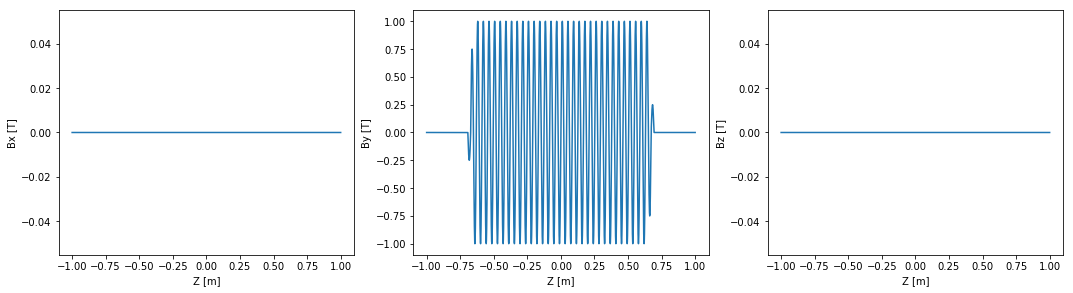## Beam¶

Add a basic beam somewhat like NSLS2. Filament beam for simple studies.

In :
# Add a basic electron beam with zero emittance
osr.set_particle_beam(energy_GeV=3, x0=[0, 0, -1], current=0.500)

# You MUST set the start and stop time for the calculation
osr.set_ctstartstop(0, 2)

# Plot trajectory
plot_trajectory_position(osr.calculate_trajectory())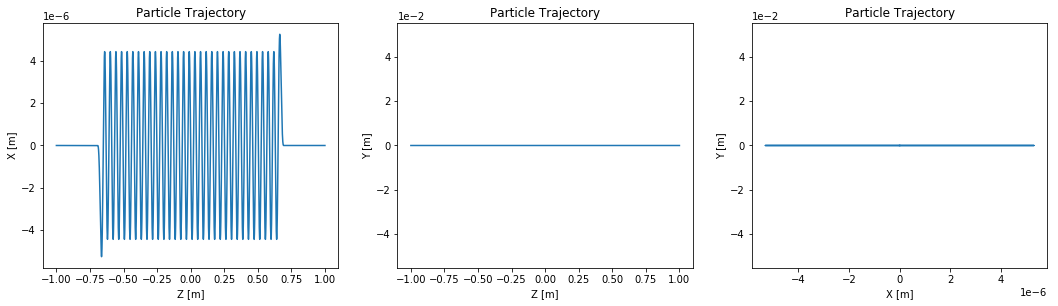## Power density - Rectangular planes¶

Calculate the power density on simple rectangular planes. The 'plane' argument is the initial plane that the surface is in. From there it can be rotated and translated. The order of 'XY' or any combination matters since the normal vector is given by X x Y (in the order written in the input).

By default the coordinates in the returned power density are relative to the center of the rectangular grid (not absolute space). If you want the coordinates in absolute space you can specify dim=3, but I give the warning that this makes plotting rotated surfaces difficult.

Note: This is the recommended method for calculating power densities on rectangular surfaces. One may also do the same with parametric surfaces (shown later in this tutorial).

In :
# Calculate power density 30 [m] downstream in the XY plane.
power_density = osr.calculate_power_density_rectangle(
plane='XY',
width=[0.030, 0.030],
npoints=[51, 51],
translation=[0, 0, 30]
)
plot_power_density(power_density)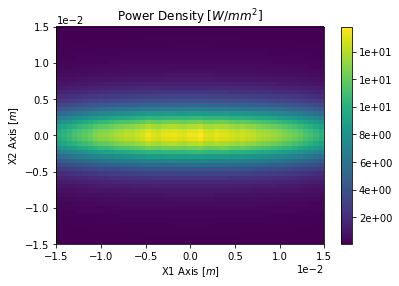In :
# We can easily rotate the above, here about the z axis.
power_density = osr.calculate_power_density_rectangle(
plane='XY',
width=[0.030, 0.030],
npoints=[51, 51],
rotations=[0, 0, osr.pi()/4],
translation=[0, 0, 30]
)

plot_power_density(power_density)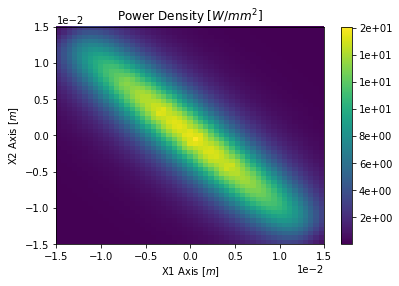In :
# Calculate the power density on a surface in the XZ plane.
# This can be thought of as the power density on the upper beampipe inner surface
power_density = osr.calculate_power_density_rectangle(
plane='XZ',
width=[0.020, 1.000],
npoints=[51, 51],
translation=[0, 0.004, 2]
)

plot_power_density(power_density)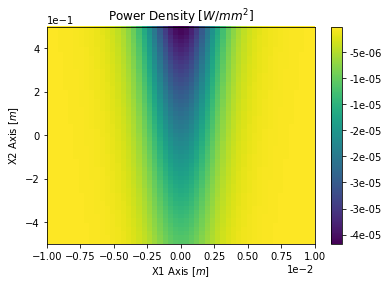In :
# We'll now take the above plane and tilt is slightly as if it were a tapered beampipe
power_density = osr.calculate_power_density_rectangle(
plane='XZ',
width=[0.020, 1.000],
npoints=[51, 51],
rotations=[0.002, 0, 0],
translation=[0, 0.004, 2]
)

plot_power_density(power_density)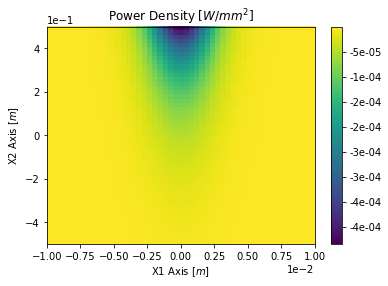## Power Density - Parametric Surfaces¶

We will now calculate the power density on a few parametric surfaces. A parametric surface in OSCARS is represented by a class. There is another tutorial which explains how to build your own.

We begin with a simple rectangular surface, then explore some others. These surfaces come from the oscars.parametric_surfaces module.

In :
# First create the surface of interest
rectangle = PSRectangle(L=0.030, W=0.030, nu=51, nv=51)

# Run calculation and plotting
pd = power_density_3d(osr, rectangle, translation=[0, 0, 30])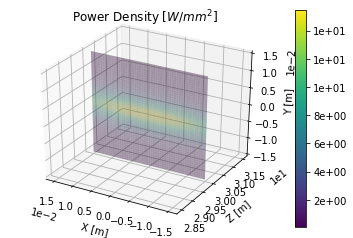In :
# It is easy to rotate the above and see it in 3D
pd = power_density_3d(osr, rectangle, rotations=[0, osr.pi()/4, 0], translation=[0, 0, 30])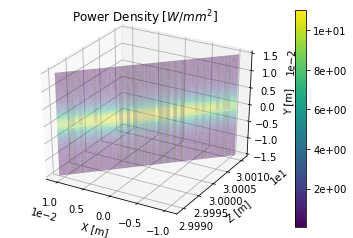In :
# First create the surface of interest
sphere = PSSphere(R=0.020, nu=51, nv=51)

# Run calculation and plotting
pd = power_density_3d(osr, sphere, translation=[0, 0, 30])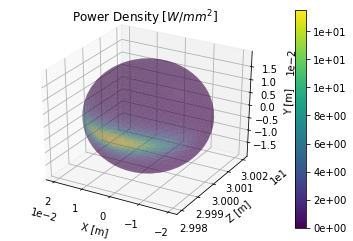In :
# First create the surface of interest
cylinder = PSCylinder(R=0.020, L=0.010, nu=51, nv=51)

# Run calculation and plotting
pd = power_density_3d(osr, cylinder, rotations=[osr.pi()/2, 0, 0], translation=[0, 0, 30])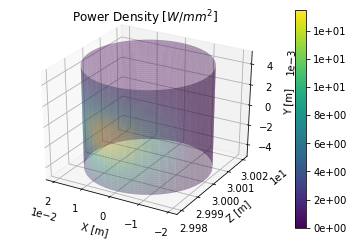In :
# Let's do a cylinder that is around the photon beam, downstream a bit
cylinder = PSCylinder(R=0.005, L=1.000, nu=51, nv=51)

# Run calculation and plotting.  Here we needed to invert the normal due to the way
# it is defined in the PSCylinder class
pd = power_density_3d(osr, cylinder, translation=[0, 0, 5], normal=-1)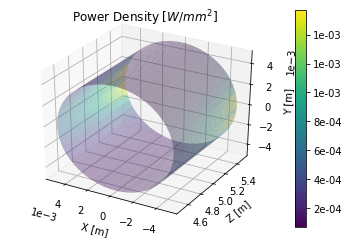## Multi-particle power density¶

It is possible to run the power density calculations in multi-particle mode in the case that you have a very large beam, or multiple beams. It is show here for completeness.

In :
# Add a basic electron beam with zero emittance
osr.set_particle_beam(
energy_GeV=3,
x0=[0, 0, -1],
current=0.500,
sigma_energy_GeV=0.001*3,
beta=[1.5, 0.8],
emittance=[0.9e-9, 0.008e-9]
)

# You MUST set the start and stop time for the calculation
osr.set_ctstartstop(0, 2)

In :
# Calculate power density 30 [m] downstream in the XY plane.
power_density = osr.calculate_power_density_rectangle(
plane='XY',
width=[0.030, 0.030],
npoints=[51, 51],
translation=[0, 0, 30],
nparticles=3
)

plot_power_density(power_density)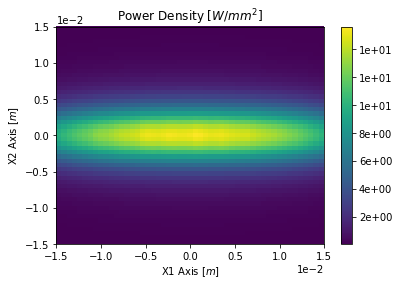## On Precision¶

The default relative precision is 0.01 (1%) and is controlled by the parameter:

• precision=0.01 (default)

You may retrieve the relative precision for all points in a calculation by including the parameter:

• quantity='precision'

Should you not reach the desired precision withing max_level you will receive a warning message. To increase max_level you have two options:

• max_level=25
• max_level_extended=(some number above max_level)

The maximum max_level is 25 due to typical memor restrictions (because it is faster). The 'extended' version runs in non-memory mode which allows higher precision at the cost of CPU time. Only in rare instances will you need this. You can also retrieve the 'level' of convergence for all points (which will show -1 for non-converged points) with the addition of:

• quantity='level'
In :
# Show the precision reached for each point.
power_density = osr.calculate_power_density_rectangle(
plane='XY',
width=[0.030, 0.030],
npoints=[51, 51],
translation=[0, 0, 30],
quantity='precision'
)
plot_power_density(power_density, title='Precision')

# Show the level reached for each point.
power_density = osr.calculate_power_density_rectangle(
plane='XY',
width=[0.030, 0.030],
npoints=[51, 51],
translation=[0, 0, 30],
quantity='level'
)
plot_power_density(power_density, title='Level')